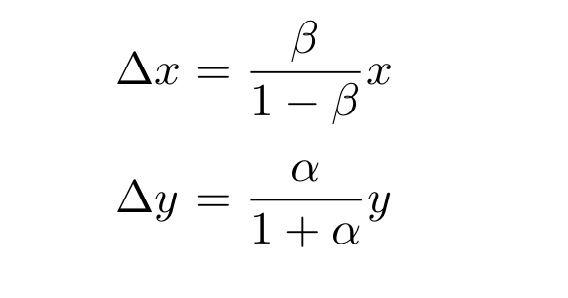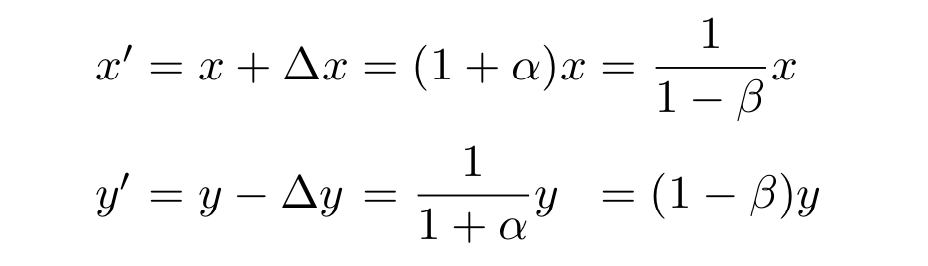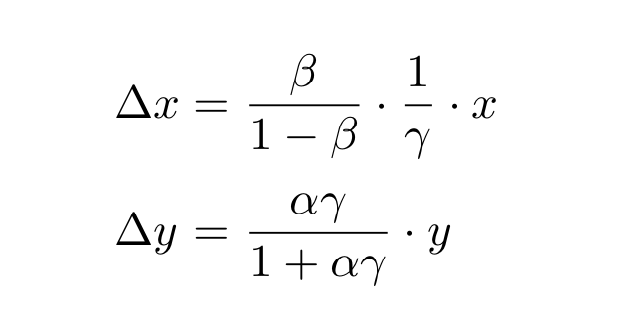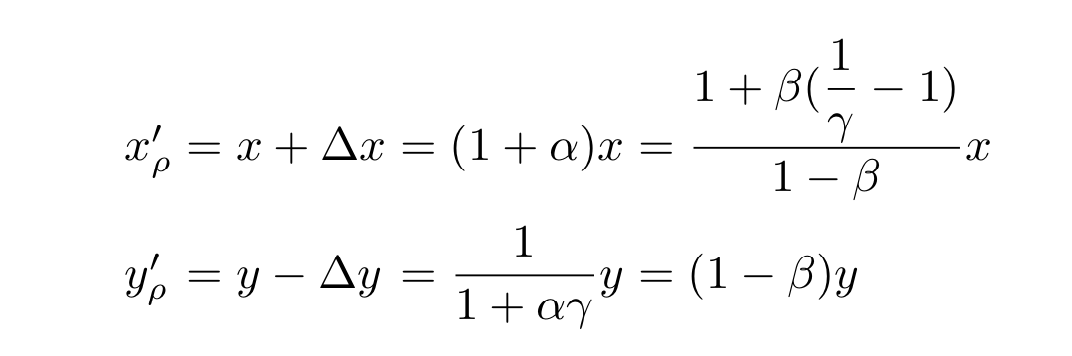# Uniwap自动化做市商核心算法解析

https://www.interchains.cc/17577.html

Uniwap自动化做市商核心算法解析是很好的区块链资料,他说明了区块链当中的经典原理，可以给我们提供资料,Uniwap自动化做市商核心算法解析学习起来其实是很简单的，

### Uniwap自动化做市商核心算法解析

• Uniswap
• 算法

Uniswap协议采用的是常量乘积做市商模型，又称为“恒定乘积做市商模型“。

## 常量乘积做市商模型

Uniswap协议采用的是常量乘积做市商模型，又称为“恒定乘积做市商模型“。数学公式表示为 x * y = k, 通过确定某一时刻x,y的兑换比例，来实现x和y之间的自动交易。所有规则定义在合约中，由区块链blockchain系统（如以太坊eth）自动执行，省去第三方做市商参与， 有时也称为：x-y-k自动做市商模型

### x-y-k模型

x-y-k模型中，x, y分别一个交易对（Pair/Pool）中某一时间两种代币的余额，x,y 对应值两种不同的代币，在目前uniswap版本中，目前只支持ERC20代币。

\$x’ = x + ∆x = (1 + alpha ) x\$ \$y’ = y – ∆y = (1 – beta) y\$

\$alpha= ∆x / x , beta = ∆y / y\$

\$ 1+alpha=frac{1}{1-beta } \$## 添加费用机制的x-y-k模型

\$x’ = x + ∆x = (1 + alpha （1 – ρ ）) * x\$

\$y’ = y – ∆y = (1 -beta ) * y\$

\$Delta x =frac{beta }{1-beta } frac{1}{1-rho } x \$

\$Delta y=frac{alpha (1-rho)}{1+alpha(1-rho )}* y\$\$ 1+beta(frac{1}{r}-1)\$

uniswap的流动性生成体现出乘积k的作用， 使用\$sqrt{k}\$ 作为流动性增长的依据，下一篇会详细说明。

## 小结

uniswap 做市商模型简单而优雅，运行近两年没有出现大的安全问题。从本节的分析中可以看到，并无高等数学积分，离散，分布等内容，高中数学水平就能理解，这也印证了简洁的力量。